# Least-squares model fitting

Last week we processed real behavioral data, generated a choice curve from it and then compared that choice curve to a softmax model. In the Assignment you messed around with the value of sigma (or equivalently beta) that you put into the softmax model until you could find the "best" value of sigma that gave a softmax curve "closest" to the data points.
Today we are going to formalize that model fitting process and get Matlab to do it automatically for us. This will allow us to automatically estimate the "best fitting" parameter value of our model.

## Reload the data and compute the choice curve

To begin copy the data into your Week_08 folder and let's create a new script that reproduces the basic choice curve from last week ...
clear
% compute expected values
EV_safe = 10;
EV_risky = P.*V;
% compute human choice curves
f_risk = mean(rsk);
f_safe = 1 - f_risk;
% plot human choice curve
clf;
plot(EV_safe - EV_risky, f_safe, '.', 'markersize', 50)
xlabel('EV_safe - EV_risky', 'Interpreter','none')
ylabel('probability of choosing safe option')
set(gca, 'fontsize', 18)
hold on;
sigma = 3;
Delta = [-6:0.1:6];
p_safe_softmax = softmax(sigma, Delta);
plot(Delta, p_safe_softmax, 'LineWidth', 3)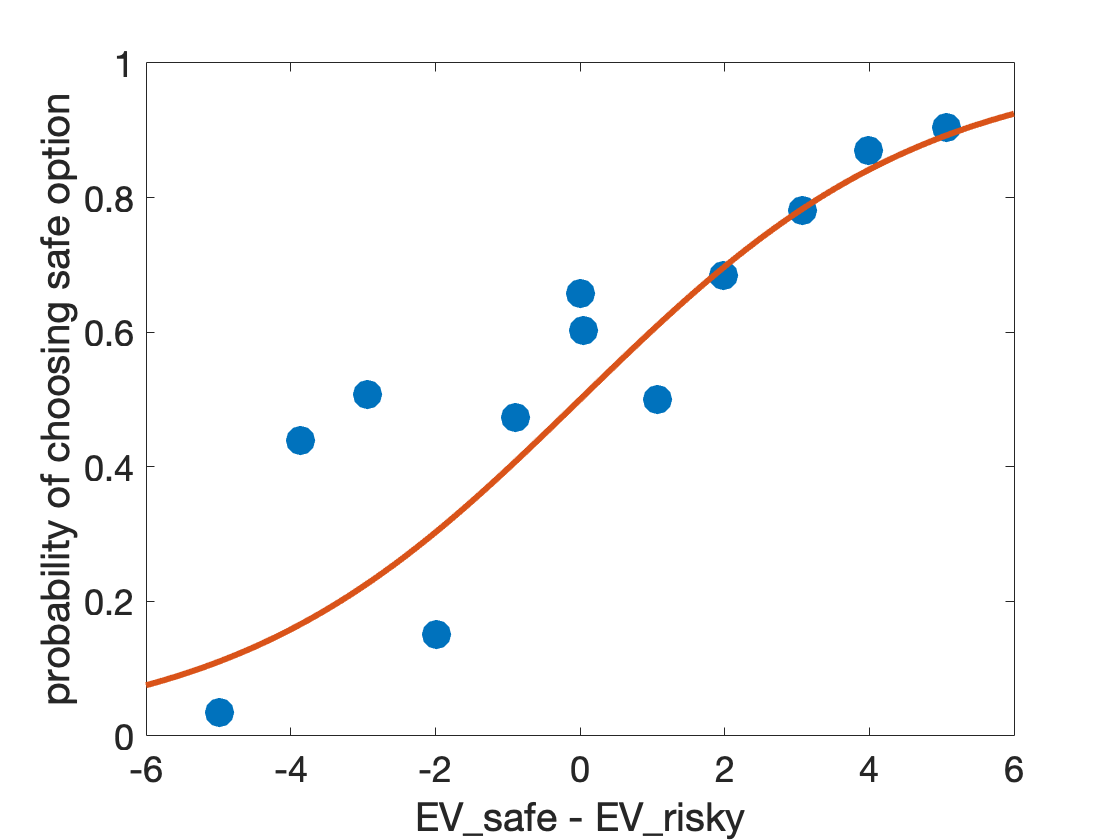## Compute the error between the model and the data

How should we quantify how well the model line fits the data? One way is to use the distance between the model line and the data points. Let's illustrate this with some code on the plot. Specifically, for each data point, I want to compute (and also plot for illustrative purposes) the distance between the data point and the line ...
% set sigma to a bad value to better illustrate errors
sigma = 1;
% recompute choice curve
p_safe_softmax = softmax(sigma, Delta);
% first replot human choice curve with the model line
clf;
plot(EV_safe - EV_risky, f_safe, '.', 'markersize', 50)
hold on;
plot(Delta, p_safe_softmax, 'LineWidth', 3)
xlabel('EV_safe - EV_risky', 'Interpreter','none')
ylabel('probability of choosing safe option')
set(gca, 'fontsize', 18)
% now go through all the data points and for each one, compute
% what the softmax value is and plot the distance on the plot
for i = 1:length(EV_risky)
modelPoint(i) = softmax(sigma, EV_safe - EV_risky(i));
plot([1 1]*(EV_safe - EV_risky(i)), [modelPoint(i) f_safe(i)], 'k-')
end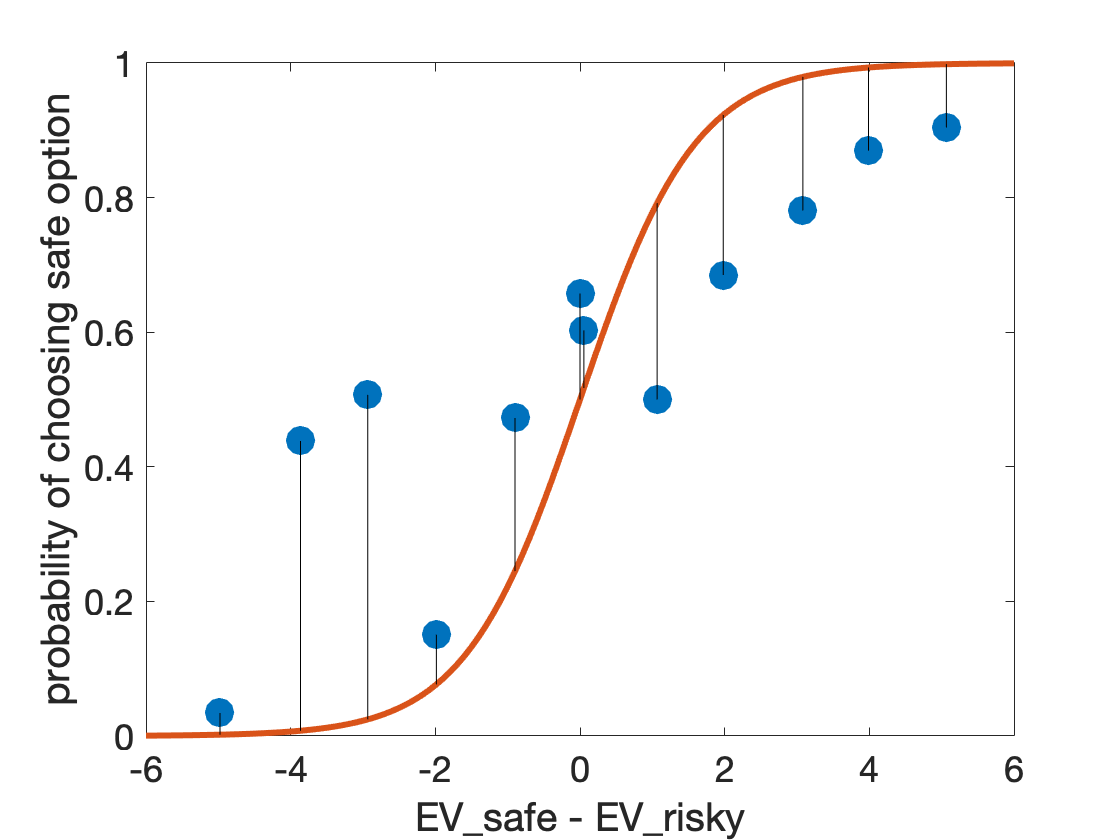The total error is something like the sum of the lengths of the black lines. We will actually use the sum of the squares of the lengths of the black lines - it doesn't really matter which for our purposes. We can compute this error like this ...
for i = 1:length(f_safe)
d = f_safe(i) - modelPoint(i);
e(i) = d^2;
end
ERROR = sum(e)
ERROR = 0.7136
Or, if we are feeling brave we can do it using element-wise raising to the power like this ...
d = f_safe - modelPoint;
ERROR = sum(d.^2)
ERROR = 0.7136
Or, if we are feeling really brave, we can go the whole hog and compute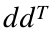for the error like this ...
d = f_safe - modelPoint;
ERROR = d*d'
ERROR = 0.7136
Either way, we get the same answer. The error is about 0.71.
Let's make a function that computes the error to hide the error code from our script ...
function ERROR = computeSumSquaredError(EV_safe, EV_risky, f_safe, sigma)
% compute values of model at data points
for i = 1:length(EV_risky)
modelPoint(i) = softmax(sigma, EV_safe - EV_risky(i));
end
% compute error
d = f_safe - modelPoint;
ERROR = d*d';
Finally, let's call the function from our script
ERROR = computeSumSquaredError(EV_safe, EV_risky, f_safe, sigma)
ERROR = 0.7136

## Computing the error for different values of sigma

So, now we know that the error when sigma = 1 is 0.7136. What does that mean? Well not very much without some more context and specifically more context about what the error is for other values of sigma. So let's use our function to compute the error as a function of sigma...
% first define some sigma values
sigma_vals = [0:0.1:10];
% then loop through all of them to compute the error
for i = 1:length(sigma_vals)
ERROR(i) = computeSumSquaredError(EV_safe, EV_risky, f_safe, sigma_vals(i));
end
% now plot the error as a function of sigma_vals
clf
plot(sigma_vals, ERROR, 'linewidth', 3)
xlabel('noise parameter, \sigma') % note the \ turns it into the Greek letter sigma
ylabel('error')
set(gca, 'fontsize', 18)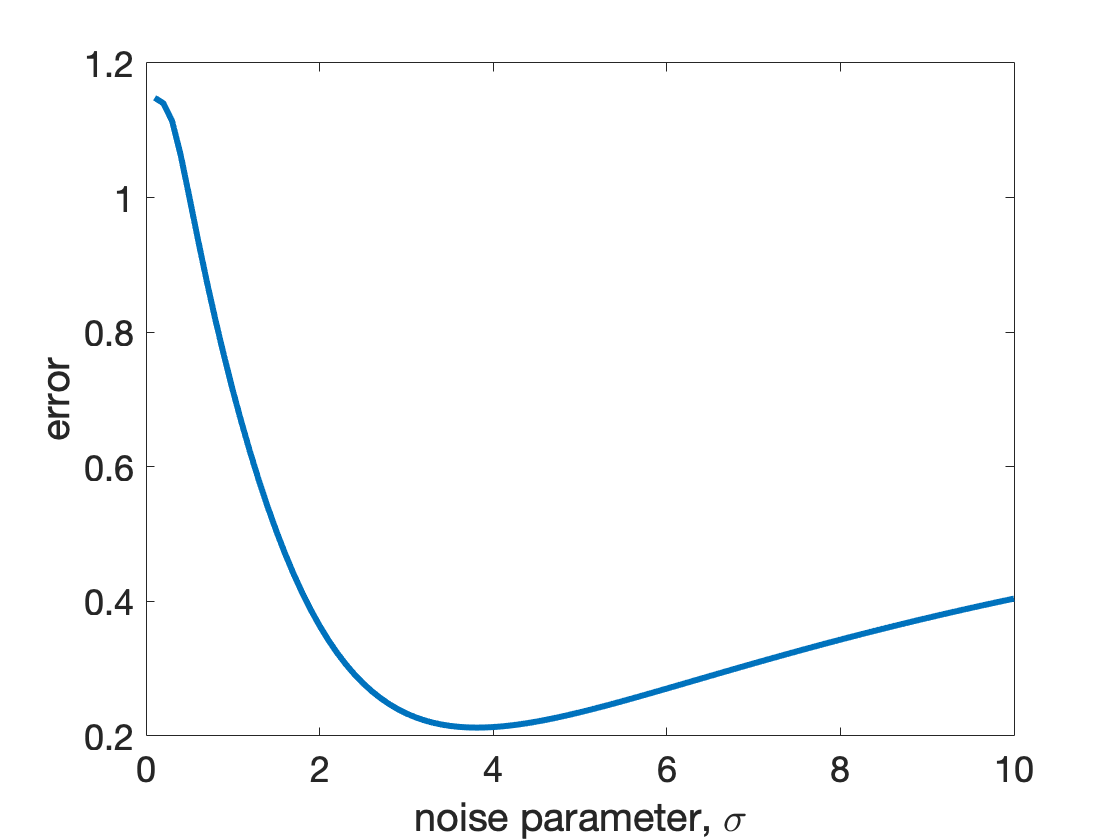So what does this tell us? Well, the error is very high for sigma = 1 and also pretty high for sigma = 10. However, there's a minimum value for the error at a particular value of sigma which seems to be just under 4. This is the best fitting value of sigma! So our guess last week of sigma = 3 was pretty close.

## Finding the minimum automatically using fmincon

OK, so we can plot out the error as a function of sigma and then use that plot to read off the best fitting value of sigma as the one that minimizes the error. Wouldn't it be nice if we could have Matlab find the minimum of the function automatically. Well it turns out that we can ...
The code to automatically find the minimum of a function revolves around something called gradient descent. The idea behind gradient descent is simple. It says that if you want to find the minimum of something you should move downhill until you can't move downhill anymore. So in our example, I might start with sigma = 1, but then I realize I'm standing on a slope so I move downhill a small amount - say to sigma = 1.1. Then I realize I'm still on a slope and move downhill again. And so on.
In this way, so long as I don't run into any problems (more on those in a minute), I'll eventually reach the bottom of the hill at the best fitting value of sigma.
At this point, you could code this gradient descent algorithm into Matlab yourself (and you will if you do the extra credit item on the Assignment), but it turns out that Matlab can do it for you with the fmincon function. However, before we can use fmincon, we first have to talk about function handles ...

## Function handles

fmincon is a general purpose function that tries to perform gradient descent on any function. However, in order for it to perform gradient descent on a function you have to actually be able to pass the function as an input into fmincon. That is you have to be able to pass a function to another function.
How you do this is not at all obvious. What I'd like to do is something like this, where I pass the computeSumSquaredError function in as an argument to fmincon
fmincon(computeSumSquaredError(EV_safe, EV_risky, f_safe, sigma))
Unfortunately if you try this you will get an error because when Matlab sees computeSumSquaredError, it tries to evaluate the function and pass the output of the function to fmincon - i.e. for one value sigma. But that's not what we want, we want to pass the whole function that fmincon can evaluate at a bunch of different sigma values to find the best fitting one.
To be able to pass a function into another function as a variable, we need a function handle. The best way to introduce a function handle is with an example.
fHandle = @(x) computeSumSquaredError(EV_safe, EV_risky, f_safe, x)
fHandle = function_handle with value:
@(x)computeSumSquaredError(EV_safe,EV_risky,f_safe,x)
This line created my function handle and assigned it to variable fHandle. The @ part tells it that what follows is a function handle, the (x) part tells it that when it sees x in the rest of the line it should treat that as an input variable (you'll see what that means in a second), then we input the function itself (computeSumSquaredError) and how we want to call it, with the EV_safe, EV_risky and f_safe all fixed but the last input (sigma) a variable, hence we write it as x.
If that doesn't make sense don't worry, because hopefully this next bit will. Once I've created my function handle I can use it like a function. For example if I write
fHandle(1)
ans = 0.7136
I get the error when sigma = 1. That is writing fHandle(1) is the SAME as writing computeSumSquaredError(EV_safe, EV_risky, f_safe, 1).
If I write
fHandle(3)
ans = 0.2335
I get the error when sigma = 3. And so on.
Unlike a pure function, though, I can pass the function handle into another function as a variable and that's exactly what I need for fmincon ...

## Back to fmincon

Now that we have our function handle we can use it as an input for fmincon. What I'd like to do at this point is just write
sigmaBest = fmincon(fHandle)
But unfortunately that will just throw an error. The reason is that when I'm doing gradient descent I need to tell the algorithm where to start. That is, I need to specify an initial value for sigma. By tradition (at least my tradition that aligns with the Matlab documentation) this initial condition is called X0. So let's set our initial value to 1
X0 = 1;
Then I can call
sigmaBest = fmincon(fHandle, X0)
Local minimum found that satisfies the constraints. Optimization completed because the objective function is non-decreasing in feasible directions, to within the value of the optimality tolerance, and constraints are satisfied to within the value of the constraint tolerance. <stopping criteria details>
sigmaBest = 3.8120
and I get a bunch of text saying a local minimum has been found and it says that sigmaBest = 3.8120 - which looks about right on our plot.
However, although this way of calling fmincon works in this case, it can be slightly better because we actually have a constraint on sigma. Specifically, we know that sigma must be greater than or equal to 0 because a negative standard deviation for the noise makes no sense.
To apply this lower bound we can define a lower bound like this
LB = 0;
And fmincon also wants an upper bound. For sigma is infinity and we can specify that like this
UB = inf;
We can then add all this into fmincon like this ...
sigmaBest = fmincon(fHandle, X0, [], [], [], [], LB, UB)
Local minimum found that satisfies the constraints. Optimization completed because the objective function is non-decreasing in feasible directions, to within the value of the optimality tolerance, and constraints are satisfied to within the value of the constraint tolerance. <stopping criteria details>
sigmaBest = 3.8120
Note: You may be wondering about all the square brackets [] in this expression. This is our way of ignoring four possible inputs to fmincon. These inputs allow us to express more complex constraints if we have more than one free parameter (e.g. we could have a constraint that one parameter must always be less than another parameter). In this class we won't use them, but such constraints do occasionally pop up in real modeling.
We can also pull out the value of the error at the best fitting value of sigma like this ...
[sigmaBest, Ebest] = fmincon(fHandle, X0, [], [], [], [], LB, UB)
Local minimum found that satisfies the constraints. Optimization completed because the objective function is non-decreasing in feasible directions, to within the value of the optimality tolerance, and constraints are satisfied to within the value of the constraint tolerance. <stopping criteria details>
sigmaBest = 3.8120
Ebest = 0.2125
And we can use this to put the best fitting point on our plot of the error ...
% now plot the error as a function of sigma_vals
clf
plot(sigma_vals, ERROR, 'linewidth', 3)
xlabel('noise parameter, \sigma') % note the \ turns it into the Greek letter sigma
ylabel('error')
set(gca, 'fontsize', 18)
hold on;
plot(sigmaBest, Ebest, '*', 'markersize', 30, 'linewidth', 3)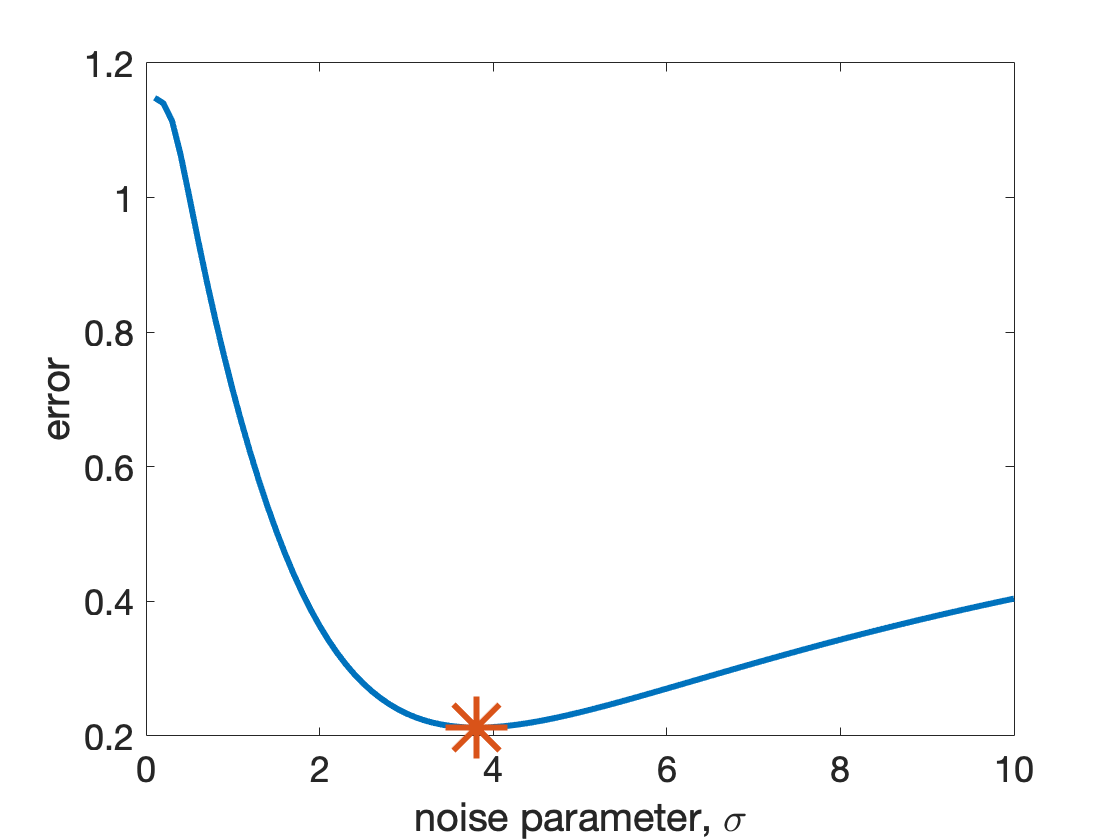As you can see, this best fitting point is right at the minimum!